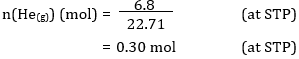# Molar Volume Formula

## How to Calculate Molar Volume of a Substance & Solved Examples

In simple words, Molar volume is the Volume occupied by one mole of any substance at a given temperature and pressure. It’s generally applied only to gases, where the identity of the gas doesn’t affect the volume. The most common example to illustrate is the molar volume of a gas at STP (Standard Temperature and Pressure), which is equal to 22.4 L for 1 mole of any ideal gas at a temperature equal to 273.15 K and a pressure equal to 1.00 atm.

In order to calculate Molar volume of a substance, we can divide the molar mass by its density. Mathematically expressing it as:
Vm=Mρ
Where, V – volume of the gas
n – Number of moles of gas, P – Pressure, T- Temperature
R – Gas Constant (value depends upon the units of Pressure, Volume and Temperature)

The gas constant R is 8.314 J / mol. K

Example: A sample of pure helium gas occupies a volume of 6.8 L at 0°C and 100 kPa.  How many moles of helium gas are present in the sample?
Options:
(a) 0.30 mol
(b) 0.15 mol
(c) 0.45 mol
(d) 0.60 mol
Solution:
V(He(g)) = volume of helium gas = 6.8 L
conditions: STP (standard temperature and pressure, 0°C and 100 kPa)
So Vm = molar volume of gas = 22.71 L mol-1Example: What is the volume occupied by 3.70 moles of N2 gas at STP?
Options:
(a) 84.0 L
(b) 50.0 L
(c) 72.5 L
(d) 22.4 L